# ISEE Middle Level Quantitative : How to find the decimal equivalent of a fraction

## Example Questions

← Previous 1

### Example Question #1 : How To Find The Decimal Equivalent Of A Fraction

Express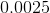as a fraction.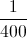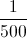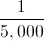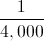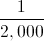Explanation:

The last nonzero digit is in the ten-thousandths place, so write the number, without decimal point or leading zeroes, over 10,000. Then reduce.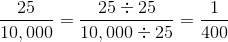### Example Question #181 : Fractions

Which is the greater quantity?

(a)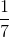(b)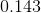(a) is greater

(a) and (b) are equal

It is impossible to tell from the information given

(b) is greater

(b) is greater

Explanation:

Divide 1 by 7: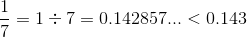### Example Question #182 : Fractions

Which is the greater quantity?

(a)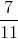(b)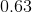(a) and (b) are equal

It is impossible to tell from the information given

(a) is greater

(b) is greater

(a) is greater

Explanation:

Divide 7 by 11: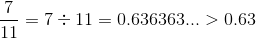### Example Question #1 : How To Find The Decimal Equivalent Of A Fraction

Which is the greater quantity?

(a)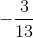(b)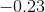It is impossible to tell from the information given

(b) is greater

(a) is greater

(a) and (b) are equal

(b) is greater

Explanation:

Divide 3 by 13: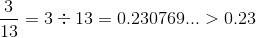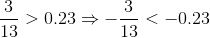### Example Question #184 : Fractions

Which is the greater quantity?

(a)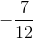(b)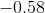(b) is greater

It is impossible to tell from the information given

(a) is greater

(a) and (b) are equal

(b) is greater

Explanation:

Divide 7 by 12: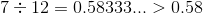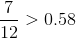, so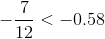### Example Question #185 : Fractions

Column A            Column B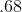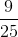The quantites are equal.

The quantity in Column A is greater.

There is no relationship between the quantities.

The quantity in Column B is greater.

The quantity in Column A is greater.

Explanation:

There are two ways to solve this problem. You can convert both to either fractions or decimals so that you can properly compare them. If you want to compare them as decimals, convert Column B to a decimal by dividing 9 by 25, which gives you 0.36. Then, you can see that Column A is greater. If you want to look at both as fractions, put 68 over 100 to get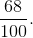This can be further simplifies toTherefore, you can see that Column A is greater.

### Example Question #186 : Fractions

Give the answer as a decimal: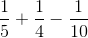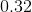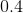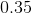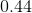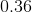Explanation: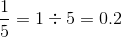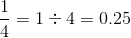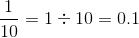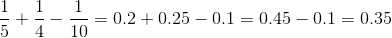### Example Question #187 : Fractions

Give the answer as a decimal: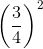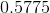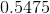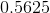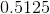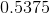Explanation: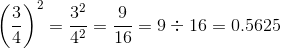### Example Question #188 : Fractions

Give the result as a decimal: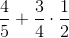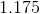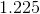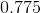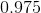Explanation: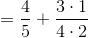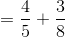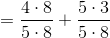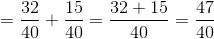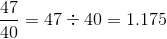### Example Question #189 : Fractions

Give the result as a decimal: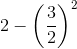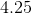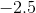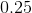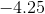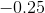Explanation:Square the fraction by squaring both the numerator and the denominator.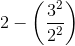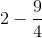To subtract, convert so that each term shares a common denominator.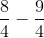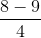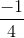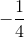Finally, convert to a decimal.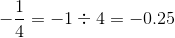← Previous 1